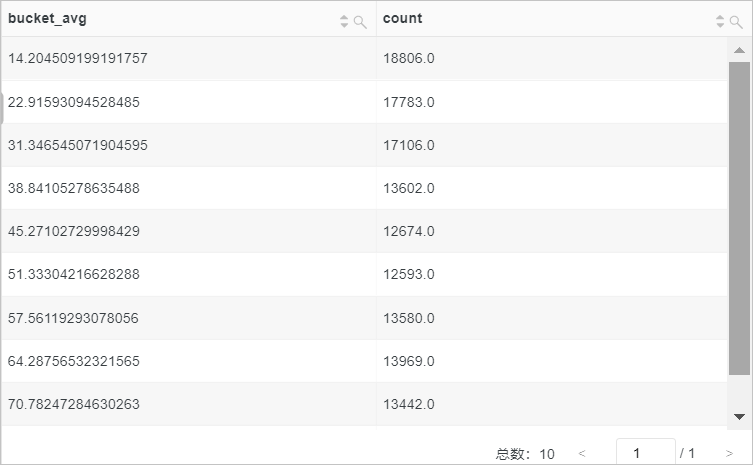approx_distinct函数 approx_distinct(x) 估算x中不重复值的个数，默认存在2.3%的标准误差。
approx_distinct(x, e) 估算x中不重复值的个数，支持自定义标准误差。
approx_percentile函数 approx_percentile(x, percentage) x进行正序排列，返回大约处于percentage位置的x
approx_percentile(x, array[percentage01, percentage02...]) x进行正序排列，返回大约处于percentage01percentage02位置的x
approx_percentile(x, weight, percentage) x和权重的乘积进行正序排列，返回大约处于percentage位置的x
approx_percentile(x, weight, array[percentage01, percentage02...]) x和权重的乘积进行正序排列，返回大约处于percentage01percentage02位置的x
approx_percentile(x, weight, percentage, accuracy) x和权重的乘积进行正序排列，返回大约处于percentage位置的x。支持设置返回结果的准确度。
numeric_histogram函数 numeric_histogram(bucket, x) 按照bucket数量（直方图列数），统计x的近似直方图，返回结果为JSON类型。
numeric_histogram(bucket, x, weight) 按照bucket数量（直方图列数），统计x的近似直方图，返回结果为JSON类型。支持对x设置权重。
numeric_histogram_u函数 numeric_histogram_u(bucket, x) 按照bucket数量（直方图列数），统计x的近似直方图，返回结果为多行多列格式。

## approx_distinct函数

approx_distinct函数用于估算x中不重复值的个数。

### 语法

• 估算x中不重复值的个数，默认存在2.3%的标准误差。
``approx_distinct(x)``
• 估算x中不重复值的个数，支持自定义标准误差。
``approx_distinct(x, e)``

### 参数说明

x 参数值为任意数据类型。
e 自定义标准误差，取值为[0.0115, 0.26]。

bigint类型。

### 示例

• 示例1：使用count函数计算PV，使用approx_distinct函数估算不重复的client_ip字段值作为UV，标准误差为2.3%。
• 查询和分析语句
``* |SELECT count(*) AS PV, approx_distinct(client_ip) AS UV``
• 查询和分析结果• 示例2：使用count函数计算PV，使用approx_distinct函数估算不重复的client_ip字段值作为UV，自定义标准误差为10%。
• 查询和分析语句
``* |SELECT count(*) AS PV, approx_distinct(client_ip,0.1) AS UV``
• 查询和分析结果## approx_percentile函数

approx_percentile函数用于对x进行正序排列，返回大约处于percentage位置的数值。

### 语法

• x进行正序排列，返回处于percentage位置的x，返回结果为double类型。
``approx_percentile(x, percentage)``
• x进行正序排列，返回处于percentage01、percentage02位置的x，返回结果为array(double,double)类型。
``approx_percentile(x, array[percentage01, percentage02...])``
• x和权重的乘积进行正序排列，返回大约处于percentage位置的x，返回结果为double类型。
``approx_percentile(x, weight, percentage)``
• x和权重的乘积进行正序排列，返回处于percentage01、percentage02位置的x，返回结果为array(double,double)类型。
``approx_percentile(x, weight, array[percentage01, percentage02...])``
• x和权重的乘积进行正序排列，返回大约处于percentage位置的x，返回结果为double类型。支持设置返回结果的准确度。
``approx_percentile(x, weight, percentage, accuracy)``

### 参数说明

x 参数值为double类型。
percentage 百分比值，取值范围为[0,1]。
accuracy 准确度，取值范围为(0,1)。
weight 权重，大于1的整数。

### 返回值类型

double类型或array(double,double)类型。

### 示例

• 示例1：对request_time列进行排列后，返回大约处于50%位置的request_time字段的值。
• 查询和分析语句
``*| SELECT approx_percentile(request_time,0.5)``
• 查询和分析结果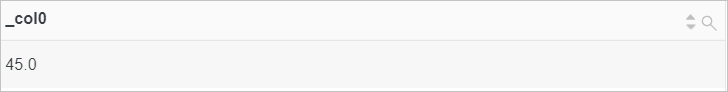• 示例2：对request_time列进行排列后，返回处于10%、20%及70%位置的request_time字段的值。
• 查询和分析语句
``*| SELECT approx_percentile(request_time,array[0.1,0.2,0.7])``
• 查询和分析结果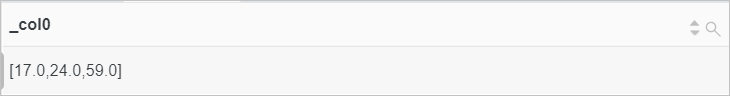• 示例3：根据request_time与权重的乘积对request_time列进行排列后，返回大约处于50%位置的request_time字段的值。其中，request_time<20时权重为100，否则权重为10。
• 查询和分析语句
``````* |
SELECT
approx_percentile(
request_time,case
when request_time < 20 then 100
else 10
end,
0.5
)``````
• 查询和分析结果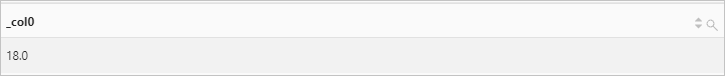• 示例4：根据request_time与权重的乘积对request_time列进行排列后，返回大约处于80%和90%位置的request_time字段的值。其中，request_time<20时权重为100，否则权重为10。
• 查询和分析语句
``````* |
SELECT
approx_percentile(
request_time,case
when request_time < 20 then 100
else 10
end,
array [0.8,0.9]
)``````
• 查询和分析结果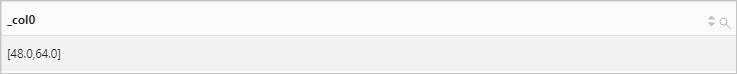• 示例5：根据request_time与权重的乘积对request_time列进行排列后，返回大约处于50%位置的request_time字段的值，准确度为0.2。其中，request_time<20时权重为100，否则权重为10。
• 查询和分析语句
``````* |
SELECT
approx_percentile(
request_time,case
when request_time < 20 then 100
else 10
end,
0.5,
0.2
)``````
• 查询和分析结果## numeric_histogram函数

numeric_histogram函数按照bucket数量（直方图列数），统计x的近似直方图。返回结果为JSON类型。

### 语法

• 按照bucket数量（直方图列数），统计x的近似直方图。
``numeric_histogram(bucket, x)``
• 按照bucket数量（直方图列数），统计x的近似直方图。支持为x设置权重。
``numeric_histogram(bucket, x, weight)``

### 参数说明

bucket 直方图中列的个数，bigint类型。
x 参数值为double类型。
weight 权重，大于0的整数。

JSON类型。

### 示例

• 示例1：统计POST方法对应的请求时长的近似直方图。
• 查询和分析语句
``request_method:POST | SELECT numeric_histogram(10,request_time)``
• 查询和分析结果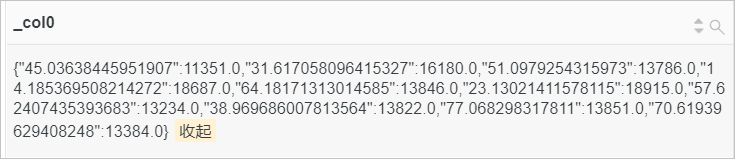• 示例2：根据request_time与权重的乘积对请求时长进行分组，从而统计POST方法对应的请求时长的近似直方图。
• 查询和分析语句
``request_method:POST| SELECT numeric_histogram(10, request_time,case when request_time<20 then 100 else 10 end)``
• 查询和分析结果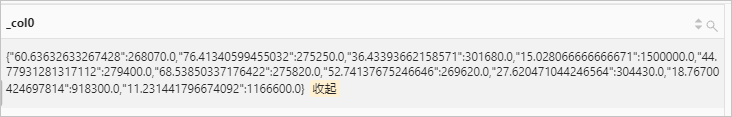## numeric_histogram_u函数

numeric_histogram_u函数按照bucket数量（直方图列数），统计x的近似直方图。返回结果为多行多列格式。

### 语法

``numeric_histogram_u(bucket, x)``

### 参数说明

bucket 直方图中列的个数，bigint类型。
x 参数值为double类型。

double类型。

### 示例

• 查询和分析语句
``request_method:POST | select numeric_histogram_u(10,request_time)``
• 查询和分析结果